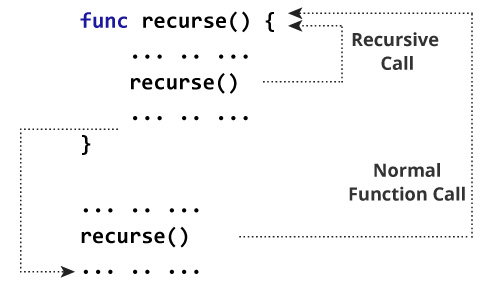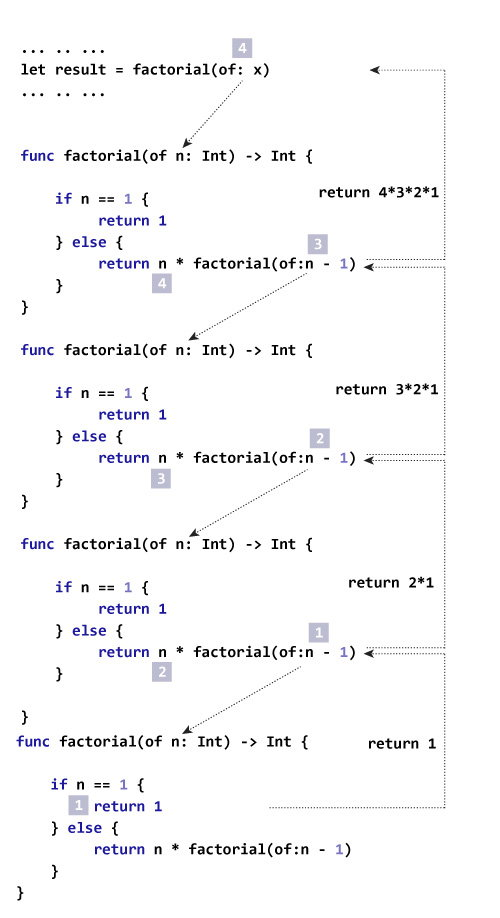# Swift Recursion

In this article, you will learn to create a recursive function; a function that calls itself.

A function that calls itself is known as a recursive function. And, this technique is known as recursion. While creating a recursive function, you must create a condition so that the function does not call itself indefinitely (infinitely).

## How recursion works in Swift?

```func recurse() {
//statements
recurse()
}
recurse()
```

The figure below shows how recursion works by calling itself over and over again.In the above flow diagram, the recursion executes infinitely. However, almost all of the times, you create a recursion that executes until some condition is met.

To prevent infinite recursion, use the recursive call inside the Swift Conditional Statements e.g. if...else statement.

## Example 1: Print N positive numbers

``````func countDownToZero(num: Int) {
print(num)
if num > 0 {
countDownToZero(num: num - 1)
}
}
print("Countdown:")
countDownToZero(num:3)
``````

When you run the following program, the output will be:

```Countdown:
3
2
1
0```

In the above program, the statement `print("Countdown:")` outputs Countdown: in the console. And the statement `countDownToZero(num:3)` calls the function that takes a parameter `Integer`.

The statement inside the function `countDownToZero()` executes and if the condition `num > 0` is met, the function `countDownToZero()` is called again as `countDownToZero(num: num - 1)`.

If condition is not met, the function call is not done and the recursion stops.

### Let's see this in steps

Execution steps
Steps Function call Printed num > 0 ?
1 `countDownToZero(3)` 3 Yes
2 `countDownToZero(2)` 2 Yes
3 `countDownToZero(1)` 1 Yes
4 `countDownToZero(0)` 0 No (Ends)

## Example 2: Find factorial of a number

``````func factorial(of num: Int) -> Int {
if num == 1 {
return 1
} else {
return num * factorial(of:num - 1)
}
}

let x = 4
let result = factorial(of: x)
print("The factorial of \(x) is \(result)")
``````

When you run the following program, the output will be:

`The factorial of 4 is 24`

### How this example works?### Let's see this in steps

Execution steps
Steps Argument passed Return statement Value
1 4 `return 4 * factorial(of:3)` 4 * factorial(of:3)
2 3 `return 3 * factorial(of:2)` 4 *3 * factorial(of:2)
3 2 `return 2 * factorial(of:1)` 4 * 3 *2 * factorial(of:1)
4 1 `return 1` 4 * 3 * 2 * 1

Usually recursion is used as a replacement of iteration when the solution to a problem can be find in about two steps. The first step searches a solution, if not repeat the process.